Email us to get an instant 20% discount on highly effective K-12 Math & English kwizNET Programs!

#### Online Quiz (WorksheetABCD)

Questions Per Quiz = 2 4 6 8 10

### Grade 4 - Mathematics8.9 Types of Fractions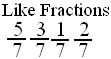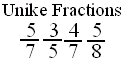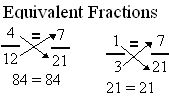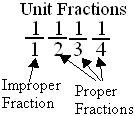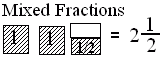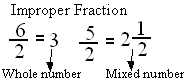Directions: Answer the following questions. Also write at least 5 examples of each type of fractions.
 Q 1: When a products obtained by cross multiplication give the same number, then the fractions are ____(or equal).mixedproperequivalent Q 2: A number with a whole number and a proper fraction is aproper numbermixed number Q 3: Fraction with numerator 1 is called a unit fraction. Are all unit fractions proper?NoYes Q 4: Fractions with same denominators are calledlike fractionsunlike fractions Q 5: If the numerator is more than the denominator in a fraction, the fraction is called a _________.proper fractionmixed fractionimproper fraction Q 6: A mixed number denotes the sum of a whole number-part and proper fraction part.TrueFalse Q 7: A fraction less than 1 is called a proper fraction. A fraction equal to one and more than 1 is called _________.proper fractionimproper fractionmixed fraction Q 8: If the numerator is less than the denominator in a fraction, the fraction is called a _______.proper fractionimproper fractionmixed fraction Question 9: This question is available to subscribers only! Question 10: This question is available to subscribers only!